# Exercise sheet 3 pdfLoading previews...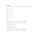partial differential equations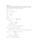partial differential equations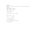partial differential equations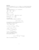partial differential equations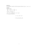partial differential equations & Lagrange's method
6 files in this resource

## Exercise sheet 3 pdf

Exercises and solutions in PDFView Item